Välj ditt land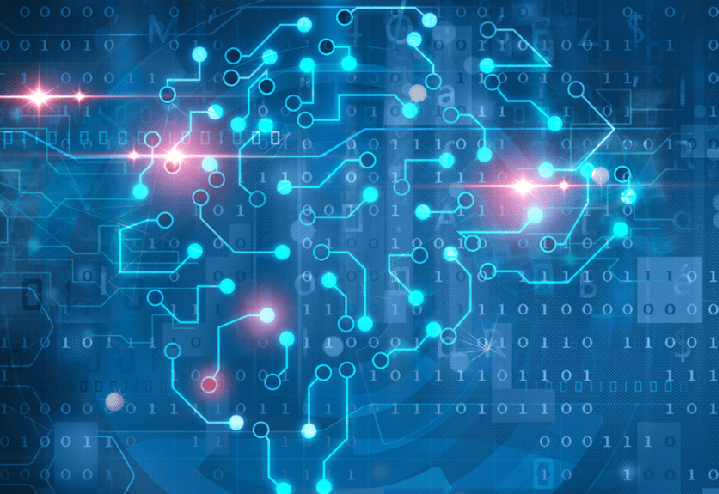# A gentle introduction to Image Recognition by Convolutional Neural Network

When people hear the words “Artificial intelligence” they most often think of either robots or automated system with the ability to distinguish between objects. The latter is what in the area of analytics knows as image recognition, a specific branch of a much wider set of techniques known as Deep Learning. When curious minds undertake the task to dig deep into the hows and whys of these techniques, they are generally overwhelmed by the mathematics involved and are too often confronted with tedious and far too technical explanations. Also, the number of examples available on the internet is rather limited (mostly due to the fact that the this branch of analytics is an emerging one). It is however possible to describe how image recognition works, or at least give a pretty good idea of it, without using overly complicated examples.

I will make an attempt at giving a clear and simple explanation of how deep learning by convolution neural networks work and end it with an example. There is nothing revolutionary about the example I have chosen. I will simply train a model to distinguish between airplane and birds. The exercice has been done before with cats, dogs, tables, chairs and so on, but it is a neat one.

My readers know by now that I do not particularily like to apply methods without really grasping the way they work and understand the math behind it. I also dislike writing about these techniques without giving something that enlightens my audience and I will therefore give a very gentle introduction to all the concepts involved in convolutional neural networks.

To do so, I will limit my example to the how the technique is used to distinguish two types of 2-dimensional images, namely those of a C and a K. The ideas can thereafter be generelized to the recognition of any letter or digits and are the same techniques used in the now so common parking lots in which an image recognition software reads your registration number, however dirty your licence plates may be.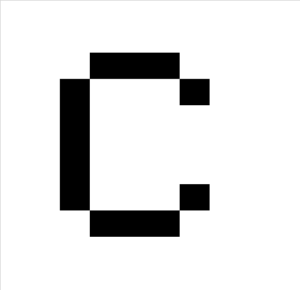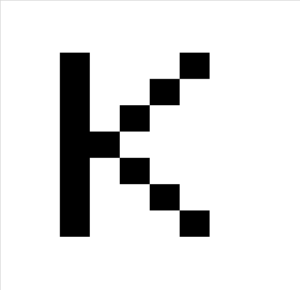As you can see, all the relevant information in these two pictures is colored black and there is no other way to distinguish them but by comparing where this information is distributed. Now, for a computer, these two images are not colored in the sense a human would understand color but rather as a 2-dimensional grid of pixels or a table in which each cell is assigned a specific number. we only have two colors here so let’s say, for the sake of the argument that the color white is -1 an color black is 1. Our computer thus translates the images as :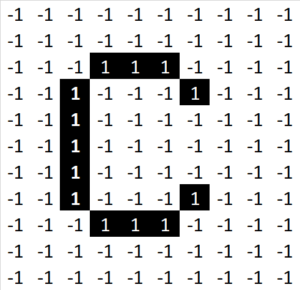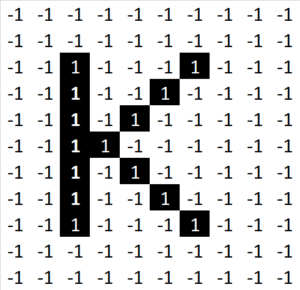Now, when comparing two pictures, if even one single pixel is different then they will be considered as different, even though they might represent exactly the same object but with different backgrounds or if the image has been somehow altered. Consider for instance the task of comparing handwritten letters (two individuals have different ways of writing an A,for instance).

This poses a serious problem if they aim is recognition of a letter or an object. So, from a machine point of view it will not be feasible to compare entire images. What we then are looking for are details or features that are common in both pictures.  Doing this task by hand is a fairly easy, but tedious, task. There is however a mathematical way to perform this task, a method that even surpasses us in classifying any set of object, both in performance and in speed. This very practical mathematical tool is called convolution. We will not into the mathematical details of convolution but will instead show how this is used in image recognition. In the picture below, we have identified one of the features specific to the letter C.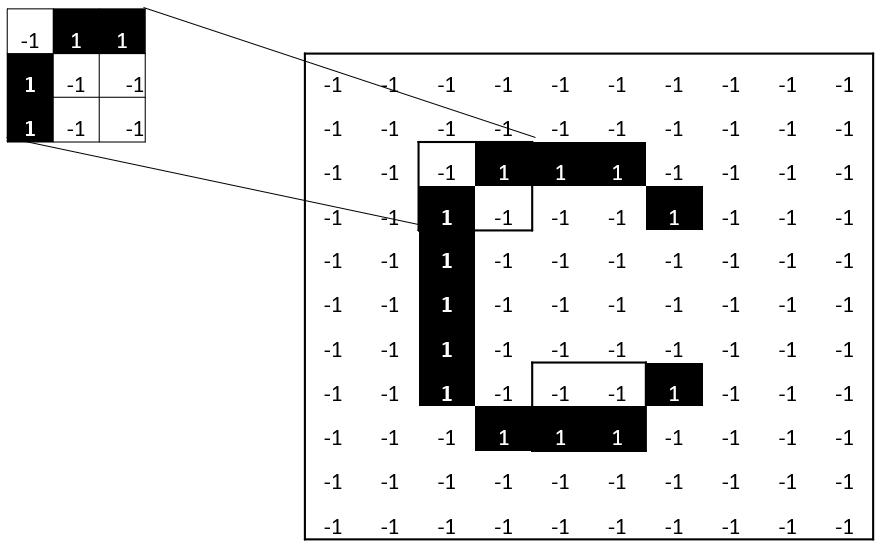When perfoming a convolution we let the identified feature (the 3 × 3 image above) sweep over the entire picture (the 10 × 11 image) and apply the following rule when the feature sweeps over a part of the image: Multiply the value of each pixel of the feature with the correpsonding pixel of the image that is covered. If both pixels have value 1 or -1, then the result is 1, otherwise the result is -1.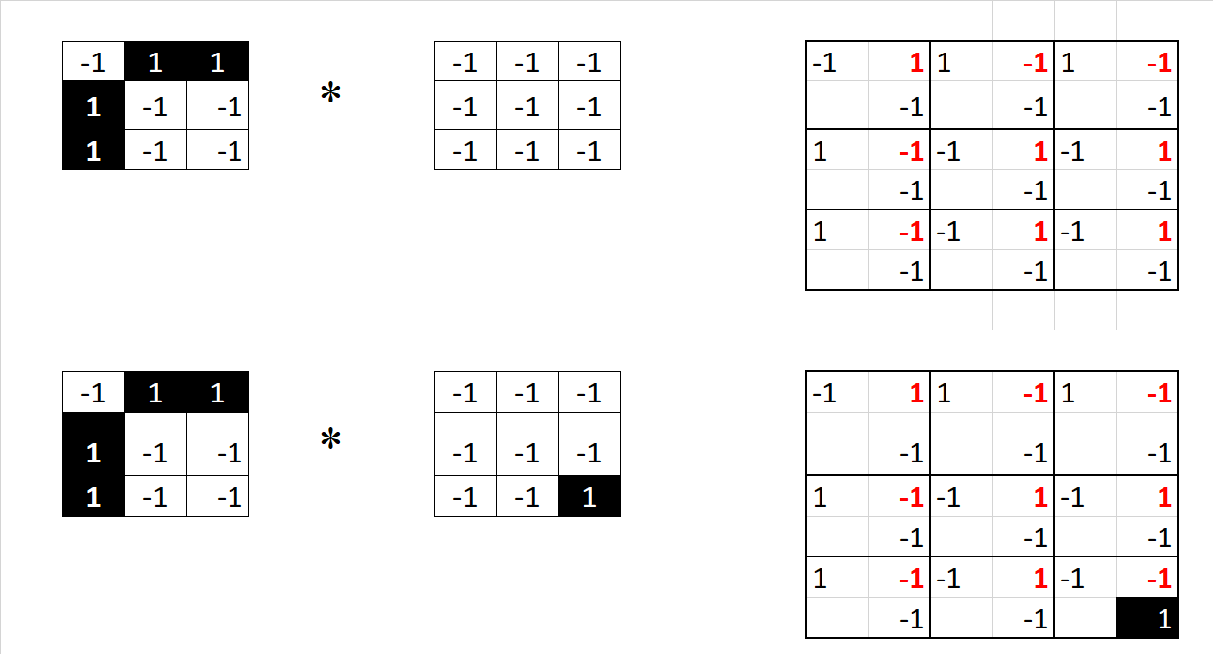The first operation represents the convolution of the feature and the upper left-hand corner of the image while the second is the convolution of the feature with the sub-part of the image starting in row 1 and column 2. The red 1s and -1s are the results on the multiplications of the corresponding pixel-values.

The next step is to add the results of the operation above and to divide the result by the total number of pixels in the feature.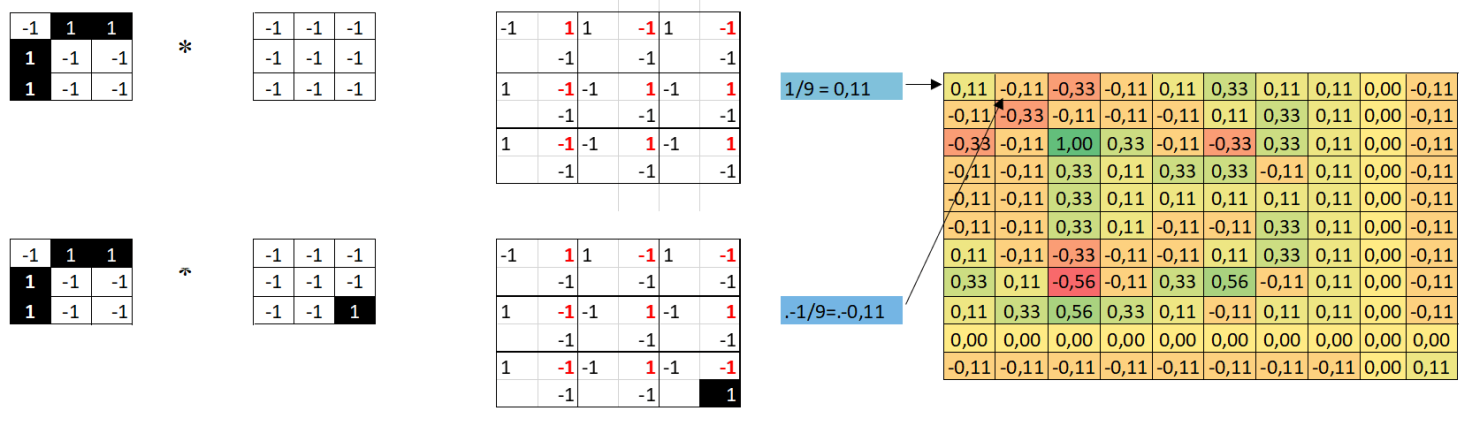The same operation then needs to be repeated for each feature of the image, the result being an array of filtered images, each one corresponding to a specific filter (feature). The set of all these operation is what we call this a convolutional layer.

A word of caution here: This is one of many convolution methods. Most often one encounters what is known as kernel convolution, where it is the center of the sub part of the image which is in consideration. Kernel convolution is used to sharpen, blur or tranform an image. I recommend the following page to learn more about these methods: AI Shack

As you might have realized by now, the number of operations needed to perform convolution operations on all images of a training set have a tendency to grow very rapidly. There are however two things one can do to limit this to something that can be handled. One, the obvious solution, is to reduce the size of the pictures to be treated. The other one if what is called max pooling which is nothing else than a downsampling of the image or, more precisely, a sample-based discretization process.

In the example I will give later on in this post I chose to reduce the size of the images as well as pooling them after convolution. So, how does pooling work? As the name indicates it’s all about keeping the essential information in the data. How is it done? Welll there are many ways of pooling data from an image depending on how much you want to reduce the image. In the picture below I given two examples, one in which the input is a 6 by 6 image reduced to a 5 by 5 image using a 2 by 2, stride 1 pooling. The second pooling is done using a 2 by 2, stride 2 sweep givning an output image of size 3 by 3.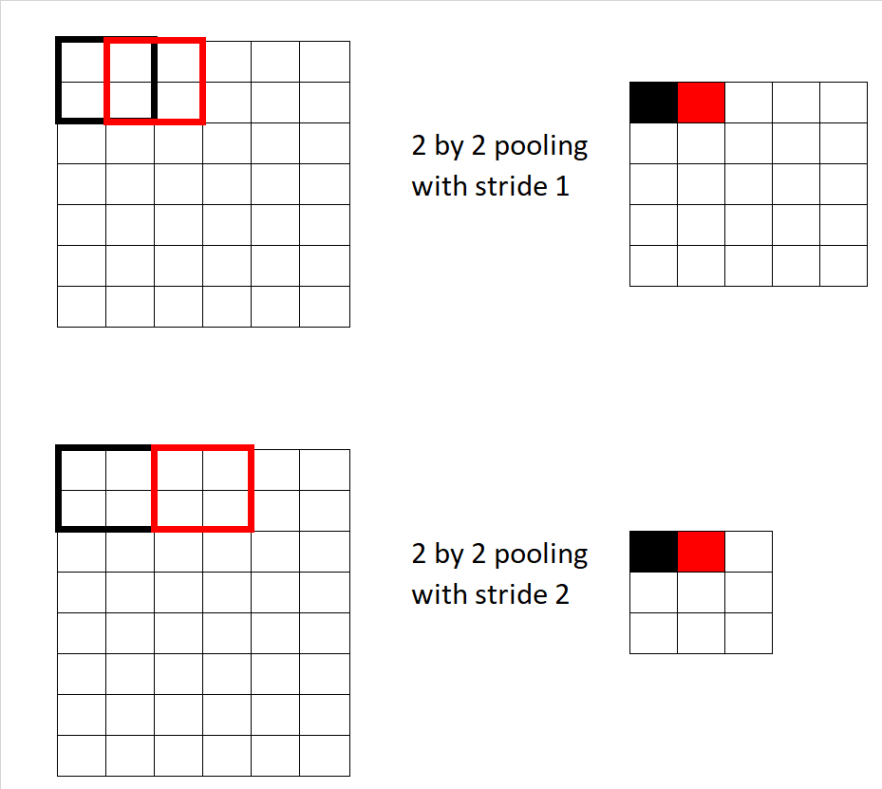If we use a 2 × 2, stride 2 pooling on our example above the resulting reduced image is given by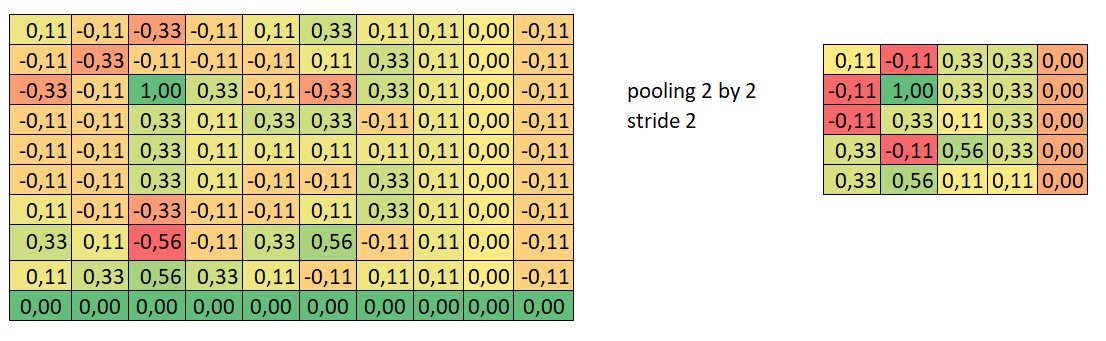having done so for all features of the convolution layer we are left with a pooling layer, a collection of heavily reduced images that are more easily treated.

The title of this post suggests the use of neural networks or neurons and until now we haven’t said anything about them. In our brain the thinking or the need to perform a specific task activates different neurons and pathways sending signals to nervs that inform muscles and other tissues to contract in a controlled manner. The neuron in a neural network is nothing else than a set of inputs, a set of weights, and an activation function.

The activation activates the neurons to perform a specific task. There are many different types of activation functions. The most common one are the sigmoidal function (most often used for classification problems of 0s and 1a), the hyperbolic tangent (used to rescale values between -1 and 1) and the Rectified Linear Unit function (ReLU) (setting all negative values to 0). Which activation function to use entirely depends on the situation one is in and we will not go into the detail in this post. To complete the example, we have been working on until now, the picture below shows the result of ReLU on the pooled and convoluted image: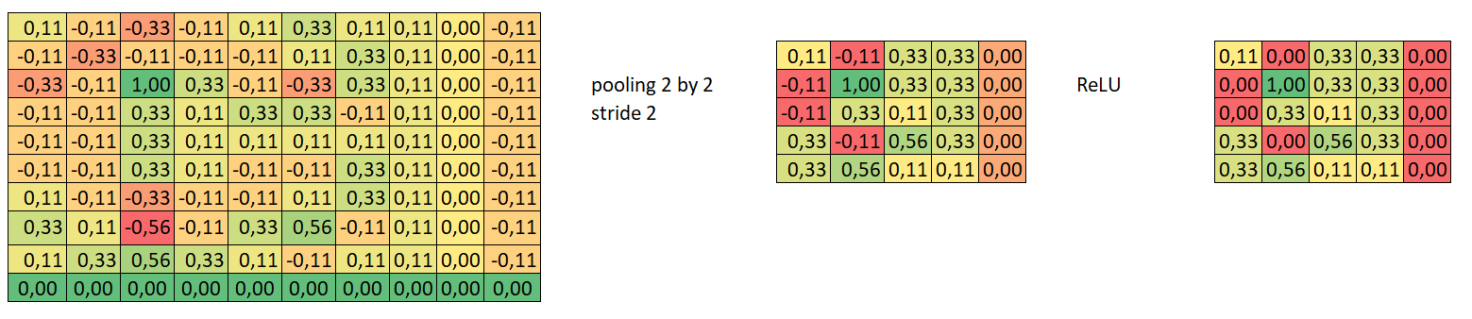The process of convoluting, pooling, activating can be repeated many times in a neural network and in this case we talk about layers. First of all we have the input layer, our pictures and last an output layer. In between, there can be one or several so called hidden layers. The output from the convolutional layers represents high-level features in the data. While that output could be flattened and connected to the output layer, adding a fully-connected layer is a (usually) cheap way of learning non-linear combinations of these features.

Essentially the convolutional layers are providing a meaningful, low-dimensional, and somewhat invariant feature space, and the fully-connected layer is learning a (possibly non-linear) function in that space. In the early days of neural networks models only included the input and output spaces (with some transformation of the data in between) and the results were found to be mediocre. It was quickly discovered that the presence of just one or two fully connected hidden layers improved the results. The entire process can be visualized as follows.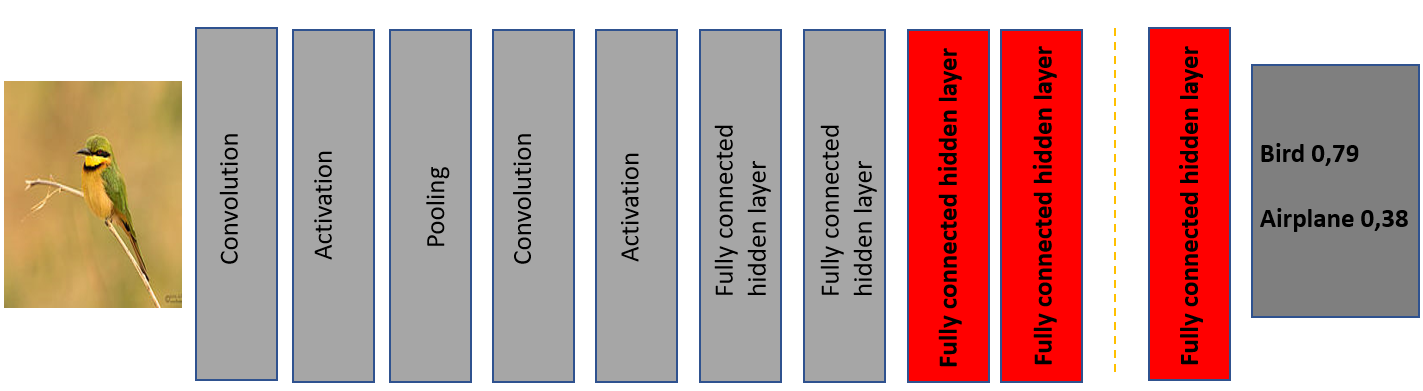This describes the entire process in a convolutional neural network. Convolutions, activations and pooling can be performed a multitude of times. Fully connected layers, the learning processes, can be added at will. The output is a probability that the object is a particular one. In this case, the probability that the input represents a “bird” is 0,79.

You now know everything there is to know about convolutions neural networks but yet lack the knowledge of making it work in practice. Let’s remedy this! As I mentioned at the beginning of this post, there is nothing revolutionary about this example. It’s been done before with different objects and has been applied IRL in many different everyday applications.

As I am a big fan of R, I have done the entire exercise using it but there are many other ways to do this. There are a few considertions to take into account before setting up your own convolutional neural network. One thing is to have enough pictures of the objects you wish to compare. In my example I have around 10 000 pics of birds and airplanes. Another more technical one which relates to the packages used is to have a later version of R. Many users of R have R 3.3.3 versions and have not updated them since they always use the same sets of packages and have no need for updates. Install at least R 3.4.0.

The packages used in the code I give below are mxnet, DiagrammeR, EBImage which need to be downloaded.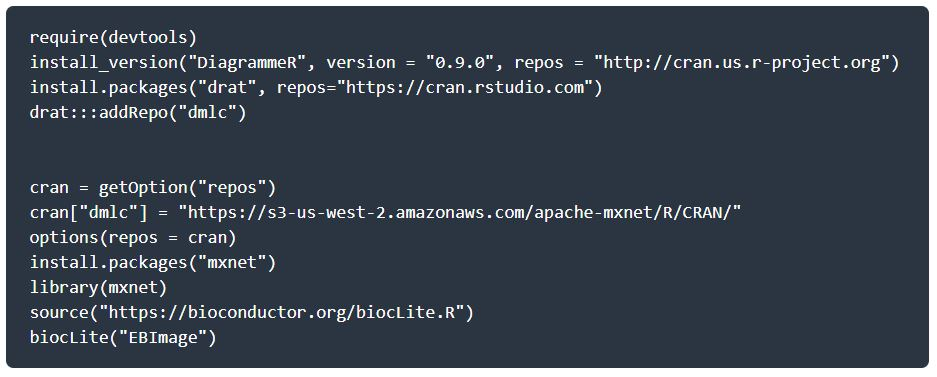Once the installation is complete, the fun begins. You need to define you working directory and point to the your image repository. Once this is done you may display images in RStudio.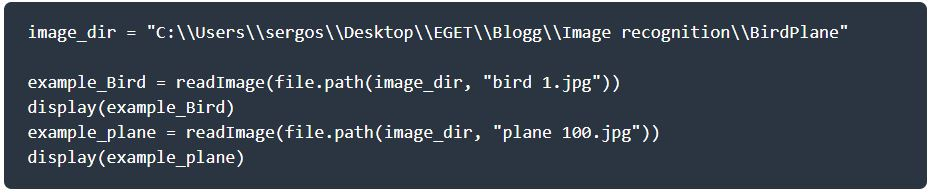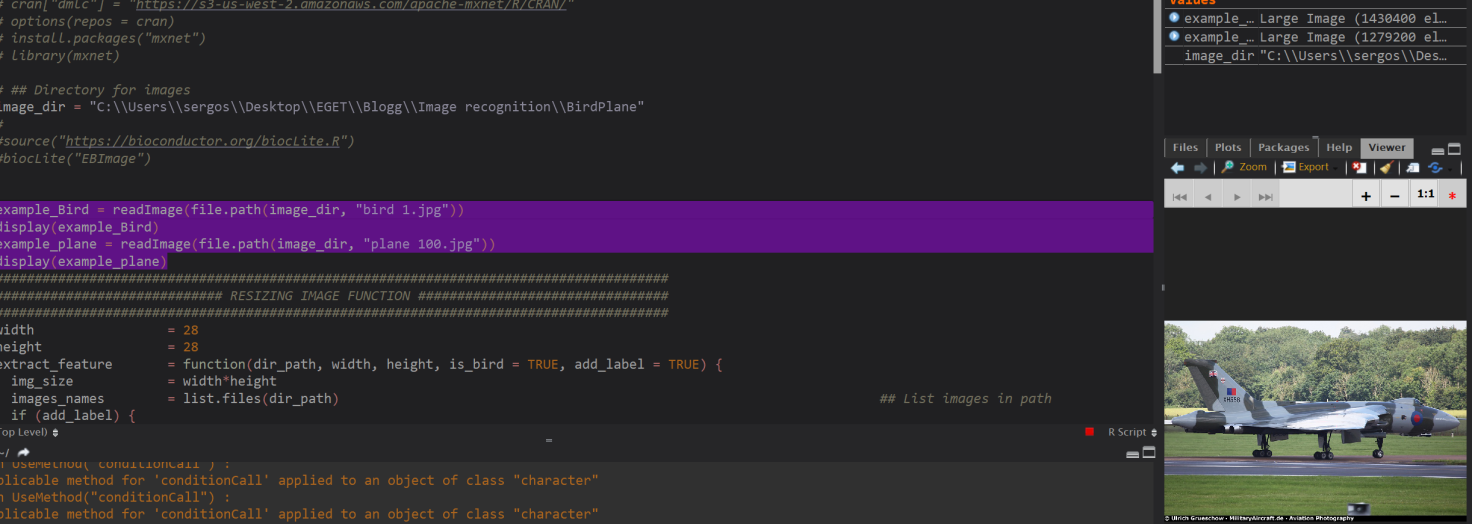How to make life easy for oneself! Most of you probably don’t have a database of pictures of the objects you wish to present to your CNN and you will most probably scrape pages in order to build a sufficient supply of images. Once you have done this, my advice is to rename all pictures. Doing this from Windows Explorer is easy but the result contains paranthesis. All bird pictures will be called bird (i), where i goes over the range of pictures you have. Use this code (saved as a *.bat file) to have the pictures renamed as bird 1, bird 2, ….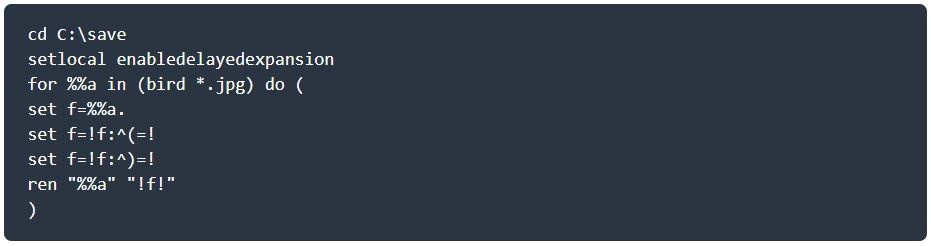As we mentioned earlier, images can take up a lot of space because of size and colors. The amount of data to be handled by the neural network can then make the whole process rather slow. It is therefore a good idea to resize the pictures and to transform them into grey scale photos (unless color is what has to be recognized as well). Here is a function that does a very good job. It grey-scales the photos and reduces the pictures to 28×28 pixels.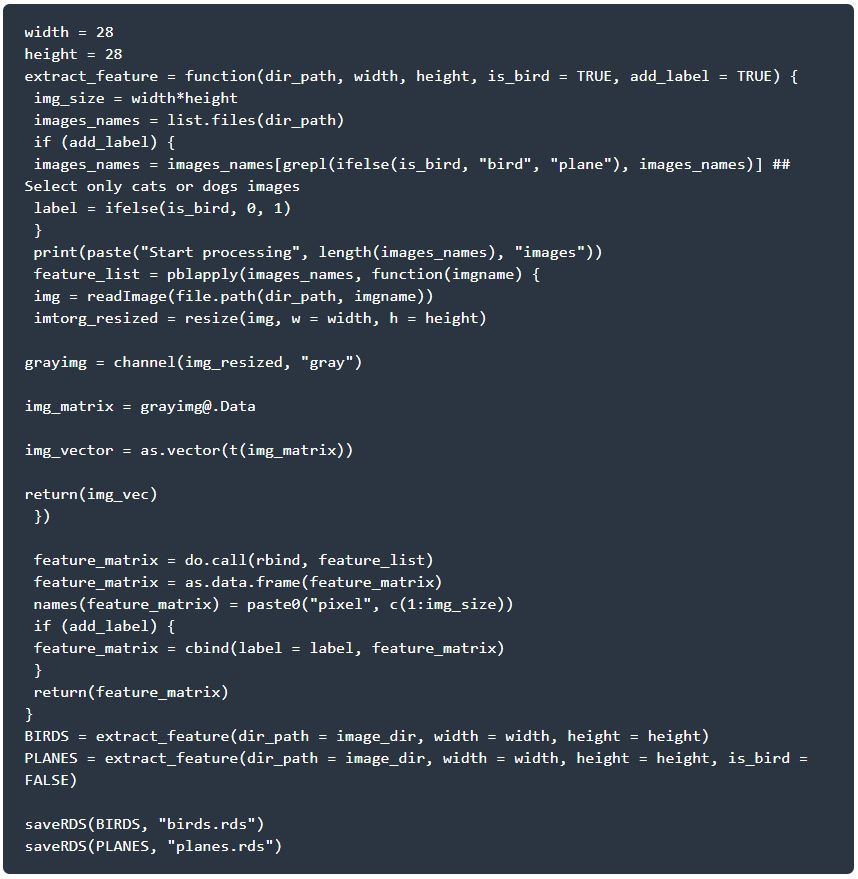We now have a library of pictures representing birds and airplanes, all pictures of the same size and color. The process above can take a while depending on the number of image to be treated, but this needs only be done onces.

The next step is to determine the training and test sets. I chose to take 60% of my photos as a training set.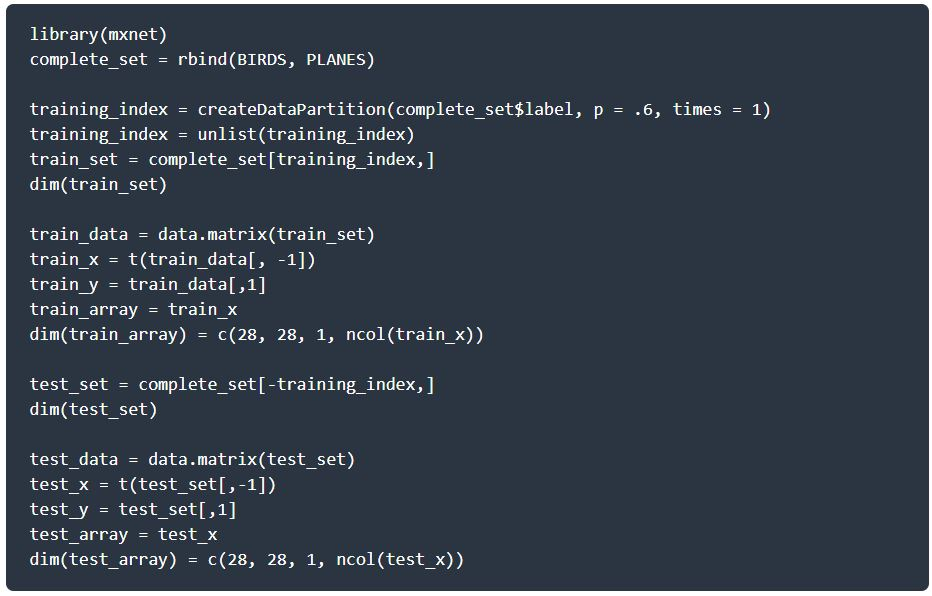Until now, we have just made some preparation of the data. We have everything set to construct our model. As we described earlier, there are several steps and ways to construct a CNN. In this example I chose to build the model by using two convolutional layer as well as two fully connected layers. Layers can be added at will depending on the purposes but one needs to keep in mind that a model with many layer can be computationally heavy to run and that the marginal gain of each added layer may not be sufficient to be motivated.

## First Convolutional Layer

In the introduction, we described how convolution could be used to identify features in an image. This was done by letting a grid sweep over the original image and perform some calculations. Furthermore, activation of neurons could be performed using some activation function and a final step was the pooling of the resulting dataset.

In this convolutional layer I use 20  5×5 grids (also called kernel) for the convolution. In the example I described above with the letters C and K, the activation function was ReLU for the simple reason that it is 1) easy to explain and 2) the image to be classified was two dimensional and not particularily complicated. However, ReLU presents some issues in this case and a far more useful and efficient activation function is the hyperbolic tangent: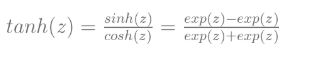For an excellent motivation of why the tanh-activation is ideal I suggest reding the following blog Derivation: Derivatives for Common Neural Network Activation Functions.

The pooling is done by taking the maximum value of the results of the activation by letting 2×2 square sweep over the the data and strides are 2 both sideways and down (hence, not overlapping).

The code for the first convolutional layer (Convolution, Activation, pooling) is then given by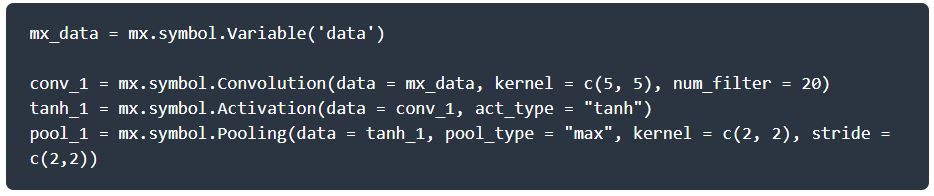## Second Convolution Layer

As i described before, one can perform multiple convolutions in order to reduce the work needed by the model and depending on the complexity of the images fed to it, it might be a good idea. In our second we refine the filtering of the image to recognize more features. Note that it is the result of the pooling in the first convolutional layer that is now used for the convolution.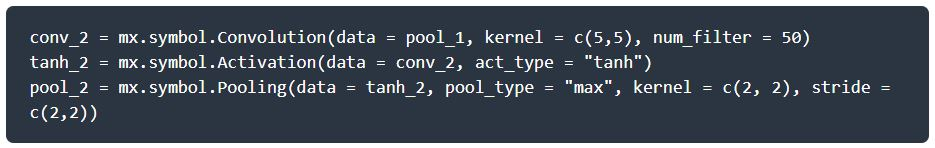## First and second Fully Connected Layers

The first fully connected Layer acts on the result of the second convolutional layer. The first step is a flattening of the results of the pooling process. The flattening is the process of converting all the resultant 2 dimensional arrays into a single long continuous linear vector.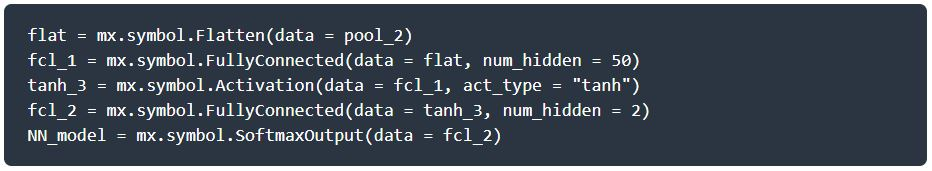The last step is jsut an activation process that assigns probabilities (i.e. values between o and 1 to the results of the second fully connected layer.

## The Model

Further up we determined a training set of pictures, defined two convolution layer and two fully connected layer. We have everything set to train our model. The mxnet package has a very good function for this, namely mx.model.FeedForward.create in which a number of parameters such as which device to use, how many rounds the model has to perform, the learning rate and so forth can be set. For a very goos documentation of the package, do read MXNet: A Scalable Deep Learning Framework.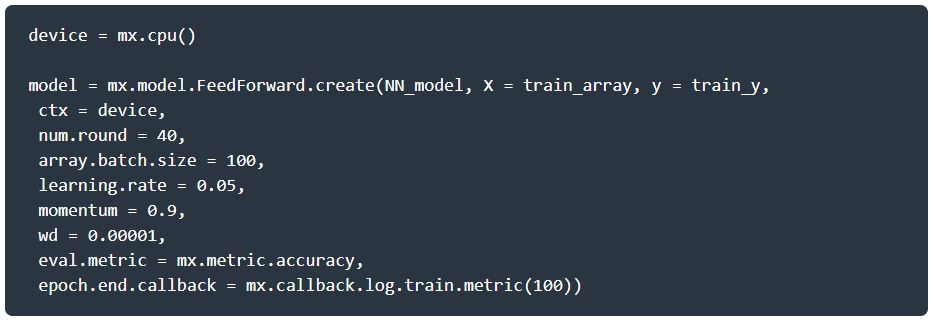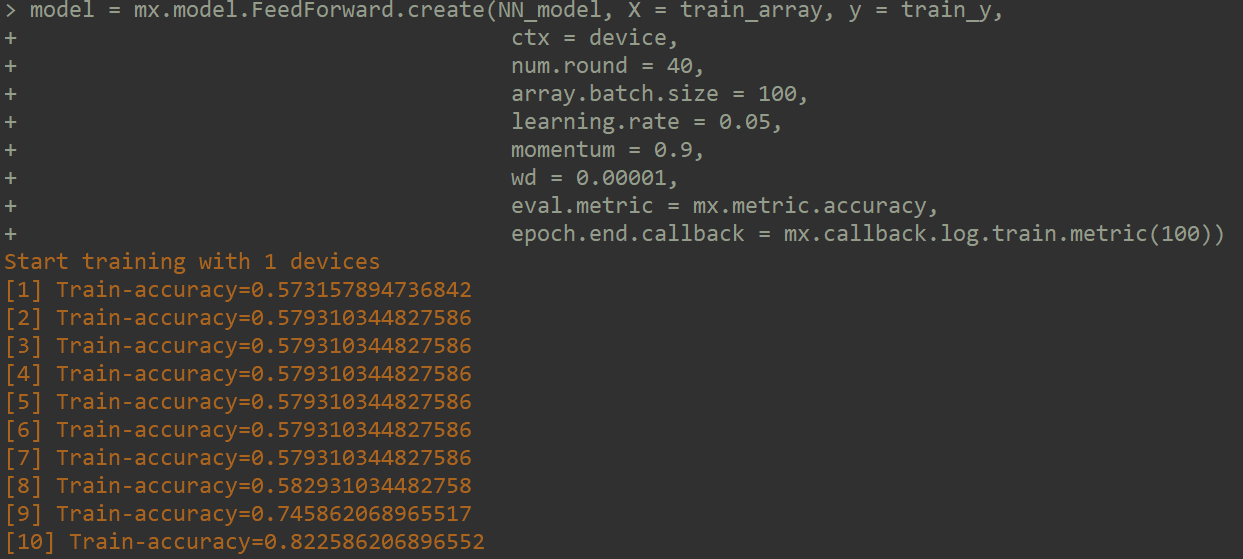In our case, the model accuracy after 40 rounds was a staggering 98,5%. For recognizing birds and airplanes, it is not a bad result. There are probably areas in which this may be too low, but let’s just say that we are failry happy with this.

We can even provide a confuction matrix for the results.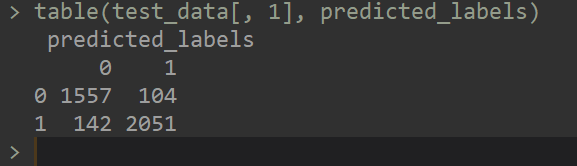As you can see, it misclassified some pictures. Just for the fun of it, here are for of the misclassified images: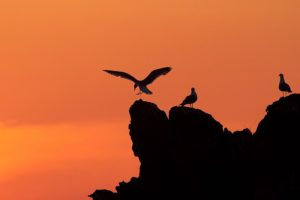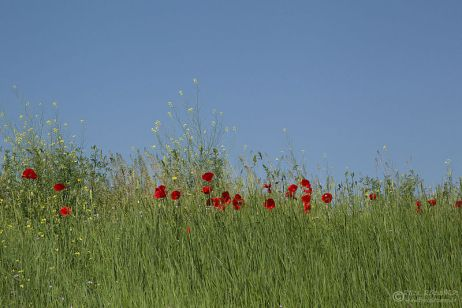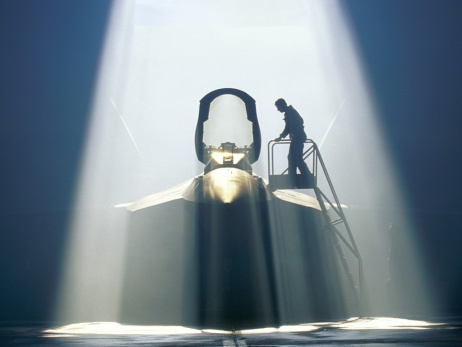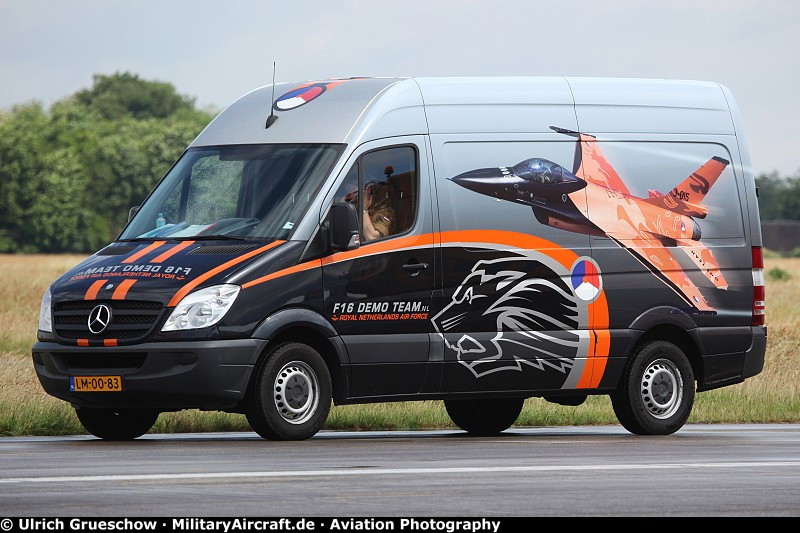These pictures are somewhat ambigious. Many other pictures that were misclassified had the same problem.

In conclusion, CNN are not very complicated and all the operations needed are fairly easy to understand. This may be one of the reasons for which we see such a widespread use of the technique.## Inläggsförfattare

• ### Serge de Gosson de Varennes

Senior Data Scientist